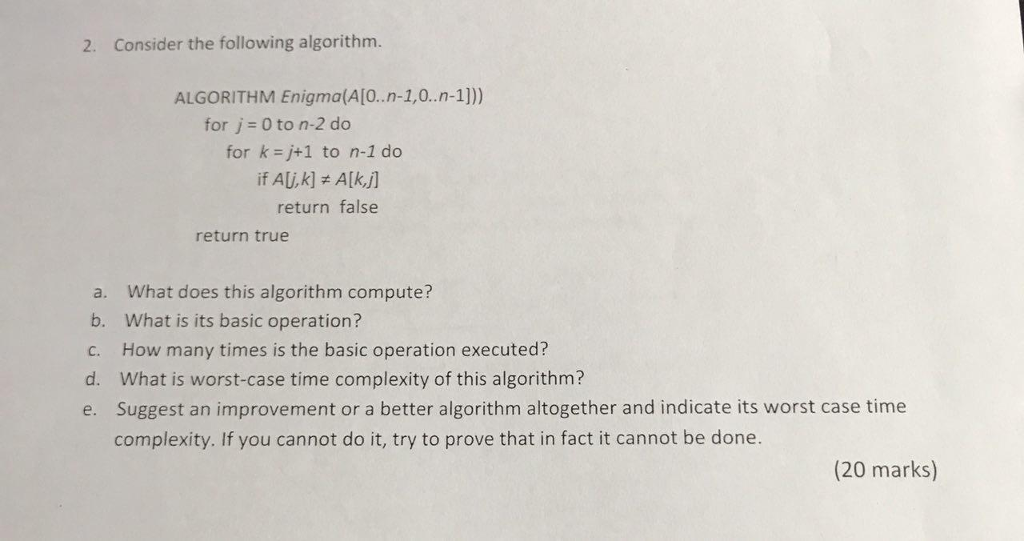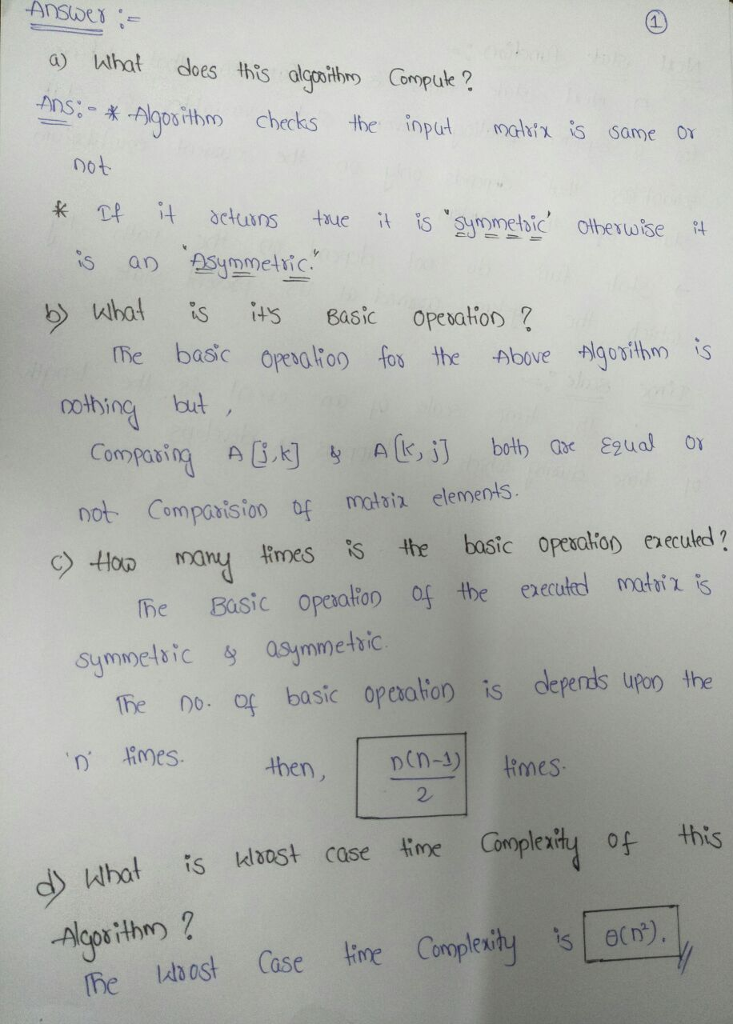# Homework Solution: Consider the following algorithm. ALGORITHM Enigma(A[0..n-l, 0..n-l))) for j = 0 to n-2 do for k = j+1 to n-1 do if A[j, k] notequa…Consider the following algorithm. ALGORITHM Enigma(A[0..n-l, 0..n-l))) for j = 0 to n-2 do for k = j+1 to n-1 do if A[j, k] notequalto A[k, j] return false return true a. What does this algorithm compute? b. What is its basic operation? c. How many times is the basic operation executed? d. What is worst-case time complexity of this algorithm? e. Suggest an improvement or a better algorithm altogether and indicate its worst case time complexity. If you cannot do it, try to prove that in fact it cannot be done.